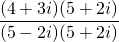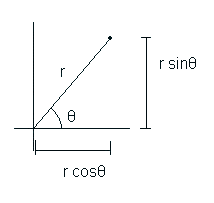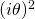# Secret NumbersI’m sure that everyone who will read this site will be familiar with the number line. Its a tool used as far back as primary school to teach children about numbersYou have been told that all numbers in existence can be represented on this one line, whether they be fractions decimals or integers. However this couldn’t be further from the truth. In reality the number line represents only a tiny fraction of all the possible numbers, and the only reason we even know about the others is because of -1, but before I get to that lets go back.

# Back to the Beginning

The number line has gone through many different variations. The first one was composed of whole positive numbers like so:It held for as long as people were doing simple stuff. If someone had 3 sheep and you gave them 5 then you could count up the number line to see they would now have eight. However as soon as subtraction was introduced thing got tricky. Sure you could do things like 5 sheep take 2 sheep, but what would happen if you had 2 and you took away 5? The way around this was to say 2-5 is the same as 0-3 (by taking 2 from each number). So 2-5=0-3 and by getting rid of the zero, 2-5=-3. And so the negative integers were born, and the number line was expanded in the other direction to account for all the nice new negative numbers.It wasn’t long however before people we once again pushing the boundaries of numbers. 4 people steal 40 cows. How many do they get each? Easy, its just 40 divided by 4 which is 10, a nice positive integer on the number line. However what if 2 people steal 1 cow? You would have to do 1 divided by 2, but there isn’t a value for that on the number line, so decimals were invented, things like 0.5, 0.25 and 0.1.But it wasn’t long before someone went and tried to divide 100 by 3. They tired and they tried but they just couldn’t do it, it wouldn’t fit on the number line as it was a never-ending number, the 3’s after the decimal point just kept going. So they did what most people would do, they didn’t bother to solve it and they invented an new way to display this infinite number, fractions! Fractions could describe not only infinite decimals but also integers and rational decimals. However there were some infinite decimals that couldn’t be expressed as fractions and these too each have their own symbols, such as,or.This is probably the extent of you’re number line knowledge, you have probably been told this is all there is to know, anything you do on the number line stays on the number line, just just move up or down depending on the operation. I’m afraid you have been misled.

# The Number That Wouldn’t FitThis is where the forbidden knowledge begins. One simple operation on one simple number with a one letter answer. But how do we solve it? The number line is just really two sets of numbers, positive and negative, whatever the number is, fraction, decimal, integer, transcendental, it is still either positive or negative, unless of course it’s zero. Now the answer forcan’t be zero,doesn’t equal -1. The number also can’t be positive as (positive number)=(positive number), and it can’t be negative as (negative number)=(positive number). So where on the number line can it go? In 1799, Caspar Wessel a mathematician decided to showon a new axis, perpendicular to the current one going through 0. The symbol forwas coined by Gauss in 1799 as i.

So no longer was there just an infinite number line, there was now an infinite number plane.And because its now a plane the numbers don’t have to stay on the axis. Just think of it like a graph, or co-ordinates. For example 1 would be (1,0) and 3i would be (0,3), but you can also have numbers like 3+2i or -4-5i, which would be at (3,2) and (-4,-5) respectivelyThis type of diagram displaying complex numbers is called an Argand Diagram.

# This Doesn’t Seem too Complex

As mentioned above you can have numbers like 4+7i or 36-21i, these are called complex numbers because they are made up of multiple parts. The general form of complex numbers isis the real part, the part that tells you how far along the real number line you go, theis the imaginary part that tells you how far up or down the imaginary number line you go. You must remember thatis a number, not two numbers added together, however, when manipulating complex numbers you do treat the real and imaginary parts separately, but it is still just one number. The following are the rules for doing things with complex numbers.

Lets say you have two complex numbers each made of a any real and any imaginary parts, like soNow if you wanted to perform an Addition of these two numbers then you would just add the real parts together and then the imaginary parts together and put the two answers back into the original form.If you wanted to perform a Subtraction with these two numbers then you just take the real numbers away from each other, then the imaginary numbers away from each other and put them back in the original formFor multiplication you can treat it like you would when expanding the brackets of a quadratic equation as shown belowYou multiply the numbers together using the acronym F.O.I.L. First.Outside.Inside.Last, so you get+++. Asis -1 thenis just. So you just group the real numbers together and do the same with the imaginary ones until you have something of the form x+iy

Now division is where it gets a bit more complex. If you have something likethen the way to solve it is to get rid of the i’s on the bottom. To do this you times the top and bottom by whatever is on the bottom, but with the sign in front of the imaginary term swapped. So you now haveNow you can focus on the top and bottom separately and then put them back together at the end, it’s just complex multiplication now. So for the top we haveand for the bottom we getPutting these back together again gives us the answer ofAs you may have noticed, no matter what operation you perform on complex numbers the form always remains the same, it’s always.

That wasn’t so hard was it? It turns out that there is a special name for the thing you times the top and bottom by. Its called the Complex Conjugate and all it is is a complex number with the sign of the imaginary part swapped, and is represented as a z with a bar over it.# Same Numbers, Different Forms

In an attempt to make life more difficult for the people learning about complex numbers, the people who had already mastered the Argand Diagram came up with a new way of expressing the same thing. And while this may not be initially good new for you, the learner, it does allow you to do some pretty awesome thing with numbers. The new way of representing them is to say that every number can be expressed as a distance from the origin and an angle from the x-axis/real axis.The bit labelled r in the diagram (the distance from the origin) is called the Modulus, and the bit labelled(the angle from the real/x axis) is called the Argument. Even though we have new names and new numbers we can still write it in the old form of,

Using some trig we can write the co-ordinates in terms of r and θ, and them sub them into the standard formIt turns out there is another way of writing complex numbers, this time with. Why they can be written in terms ofis relatively straightforward and is shown below. First of we have the expansion of e as an infinite series of addition (for all those interested this is done using the Taylor expansion)We then replacewith. Now because the‘s are being raised to powers sometimes the‘s will become 1, -1 ore.giswhich isWe now group all of the terms withtogether and all those withouttogether, like soNow these bits in brackets bear a striking resemblance to the infinite series expansions of Sin and CosTa-Da, done. We now have a new way of expressing complex numbersAnd if the modulus of the number is anything other than 1 we can writeWriting complex numbers in this form the Argument (angle) and Modulus (distance) are called Polar Coordinates as opposed to the usual (x,y) Cartesian coordinates.

# Magic e

When it comes to complex numbers,lets you do complex operations with relative ease, and leads to the most amazing formula in all of maths. As before there are special ways you manipulate complex numbers in this form.

For Addition and Subtraction in theform its best to just convert them back to theform. It makes your life a lot easier and save you loads of horrible trig and geometry.

For Multiplication you just times the two moduli together and add the two angles togetherand for Division you just divide the two moduli and minus the two anglesWriting complex numbers in terms of its Polar Coordinates allows ALL the roots of real numbers to be calculated with relative ease. To calculated theroot of a number a you just use the following formulaWhen you take the nth root a number you get n answers all lying on a circle of radius n√a, with the roots being 360/n° apart. This may all sound confusing so hopefully these two diagrams will help. Here we have all three cube roots of 1and here are all five of the 5th roots of 6Its using the ability to put complex numbers in this form that gives rise the the most beautiful and amazing identity in Maths.

# The Most Amazing Identity in all of MathsYou get the identity by putting θ in as πr (which is equal to 180°) and then adding one. In graphical terms the identity looks like this

Its heralded as the greatest identity in maths because it contains the following numbers

• The number 0.
• The number 1, which is unity. It is the multiplicative identity of the integers and the real and complex numbers
• The number π, which is found all over especially in trigonometry, geometry, and mathematical analysis. Has a decimal length of ∞
• The number e, the base of natural logarithms, which also occurs widely in mathematical analysis. Has a decimal length of ∞
• The number i, imaginary unit of the complex numbers, which contain the roots of all non-constant polynomials.

The great physicist Richard Feynman said of the equation that it’s “the most remarkable formula in mathematics”, for its single uses of the notions of addition, multiplication, exponentiation, and equality, and the single uses of the important constants 0, 1, e, i and π.